# 几种常见的损失函数

## 1. 损失函数、代价函数与目标函数

损失函数（Loss Function）：是定义在单个样本上的，是指一个样本的误差。
代价函数（Cost Function）：是定义在整个训练集上的，是所有样本误差的平均，也就是所有损失函数值的平均。
目标函数（Object Function）：是指最终需要优化的函数，一般来说是经验风险+结构风险，也就是（代价函数+正则化项）。

--

## 2. 常用的损失函数

这一节转载自博客

### （1）0-1损失函数（0-1 loss function）

L(y,f(x))={1,0,y≠f(x)y=f(x)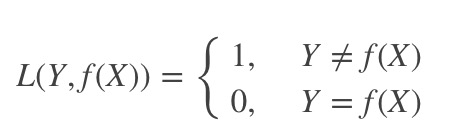也就是说，当预测错误时，损失函数为1，当预测正确时，损失函数值为0。该损失函数不考虑预测值和真实值的误差程度。只要错误，就是1。

L(y,f(x))=(y−f(x))2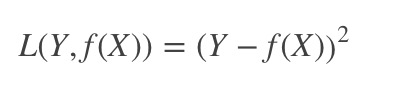是指预测值与实际值差的平方。

### （3）绝对值损失函数（absolute loss function）

L(y,f(x))=|y−f(x)|L(y,f(x))=|y−f(x)|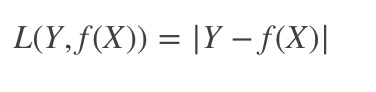该损失函数的意义和上面差不多，只不过是取了绝对值而不是求绝对值，差距不会被平方放大。

### （4）对数损失函数（logarithmic loss function）

L(y,p(y|x))=−logp(y|x)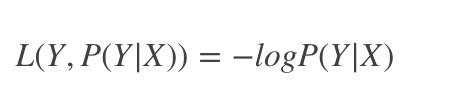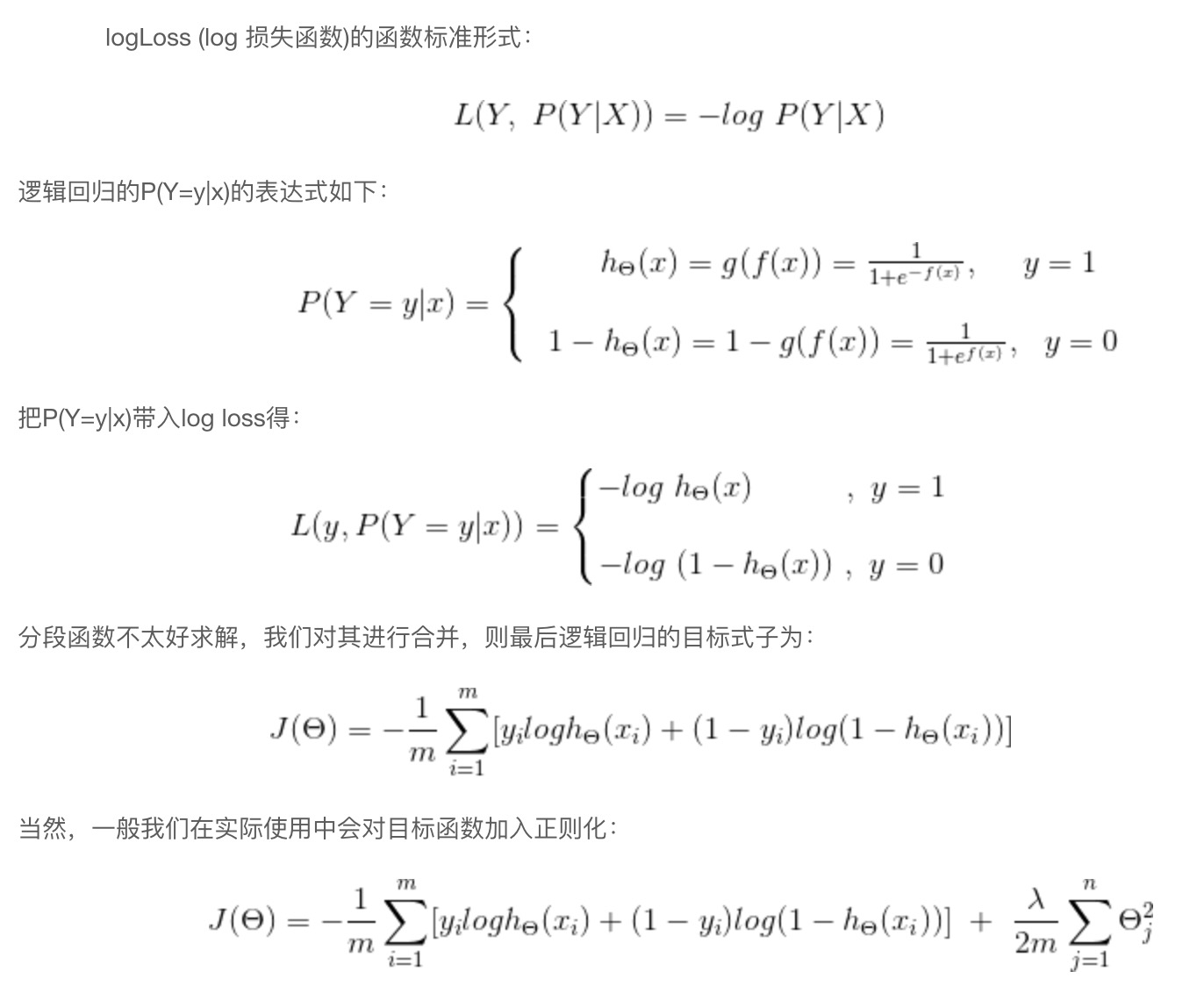这个损失函数就比较难理解了。事实上，该损失函数用到了极大似然估计的思想。P(Y|X)通俗的解释就是：在当前模型的基础上，对于样本X，其预测值为Y，也就是预测正确的概率。由于概率之间的同时满足需要使用乘法，为了将其转化为加法，我们将其取对数。最后由于是损失函数，所以预测正确的概率越高，其损失值应该是越小，因此再加个负号取个反。

### （5）Hinge loss

Hinge loss一般分类算法中的损失函数，尤其是SVM，其定义为：

L(w,b)=max{0,1−yf(x)}L(w,b)=max{0,1−yf(x)}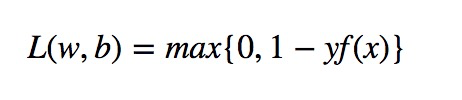其中 y=+1或y=−1 ，f(x)=wx+b ，当为SVM的线性核时。

（6）指数损失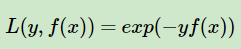## 3. 常用的代价函数

### （1）均方误差（Mean Squared Error）

MSE=1N∑i=1N(y(i)−f(x(i)))MSE=1N∑i=1N(y(i)−f(x(i)))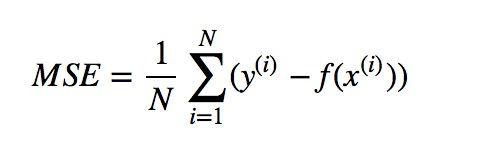均方误差是指参数估计值与参数真值之差平方的期望值; MSE可以评价数据的变化程度，MSE的值越小，说明预测模型描述实验数据具有更好的精确度。（ ii 表示第 ii 个样本，NN 表示样本总数）
通常用来做回归问题的代价函数

### （2）均方根误差

RMSE=1N∑i=1N(y(i)−f(x(i)))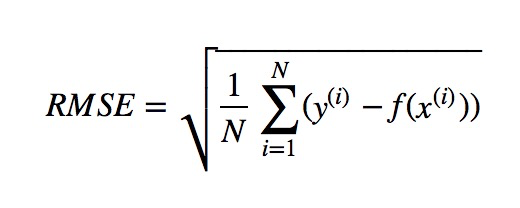均方根误差是均方误差的算术平方根，能够直观观测预测值与实际值的离散程度。
通常用来作为回归算法的性能指标

### （3）平均绝对误差（Mean Absolute Error）

MAE=1N∑i=1N|y(i)−f(x(i))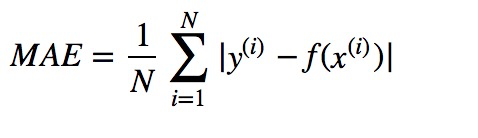平均绝对误差是绝对误差的平均值 ，平均绝对误差能更好地反映预测值误差的实际情况。
通常用来作为回归算法的性能指标

### （4）交叉熵代价函数（Cross Entry）

H(p,q)=−∑i=1Np(x(i))logq(x(−i))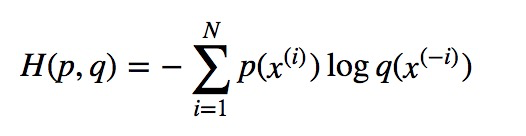交叉熵是用来评估当前训练得到的概率分布与真实分布的差异情况，减少交叉熵损失就是在提高模型的预测准确率。其中 p(x)p(x) 是指真实分布的概率， q(x) 是模型通过数据计算出来的概率估计。
比如对于二分类模型的交叉熵代价函数（可参考逻辑回归一节）：

L(w,b)=−1N∑i=1N(y(i)logf(x(i))+(1−y(i))log(1−f(x(i))))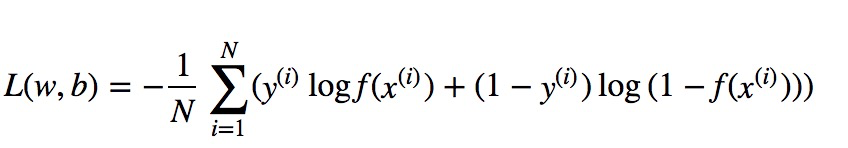其中 f(x)可以是sigmoid函数。或深度学习中的其它激活函数。而 y(i)∈0,1 。
通常用做分类问题的代价函数。